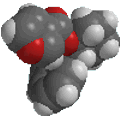Chapter 1: Structure Determines Properties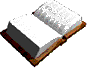Representing 3D Molecular Shapes in 2D:

The JSMOL image below shows an interactive model of a molecule of methane, CH4 (you can use the mouse to turn the molecule). Figure I shows how this 3D image would be represented on a piece of paper.  Unbroken lines are used to represent bonds in the plane of the paper, hashed wedges represent bonds going back out of the plane of paper and solid wedges represent bonds coming out of the plane of the paper. See if you can manipulate the molecule in the Chime image below to match the drawing in figure II.

 CH4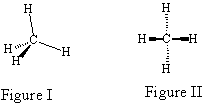SN2 (later) reactions go via a trigonal bipyramidal transition state. The structures below are from the reaction of ethyl bromide with water, CH3CH2Br + H2O. Can you manipulate the trigonal bipyramidal transition state , given in the 3D JSMOL image below, so that it matches the drawings in each of the figures immediately to the right?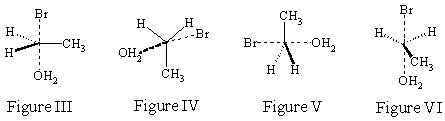C2H7OBr
Notice that bonds that are in the process of being formed or broken are given as dashed lines. The representations that are clearest then are those that place those bonds in the plane of the paper so that they do not get confused with the dashed wedges that represent bonds coming out of the plane of the paper (Figure I  III).

The most desirable drawings are usually the simplest, generally they place as many atoms as possible in the plane of the paper.

Now consider the molecule n-butane, JSMOL image given below.  Manipulate the molecule so that it matches the drawing in Figure IX.

 C4H10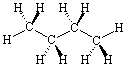Figure IX

So what happens when lone pairs of electrons are involved?  The most desirable drawings still place the most bonding sites in the plane of the paper even though lone pairs of electrons are most easily represented as being in the plane of the paper (it is not easy to wedge and hash a lone pair).

Consider the JSMOL image for NH3 given below.  Figure X would be the best drawing since it considers the lone pair on nitrogen as being in a bonding site in the plane of the paper.  As you can see in Figure XI it is much more confusing if you choose to represent the lone pair as coming out of the paper.

 NH3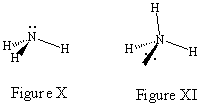Ethyl methyl ether is a structure where the opposite is true.  Drawing the lone pairs in the plane of the paper would be far more confusing than if you consider them as being out of the plane.

 C3H8O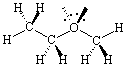Figure XII

You need to learn to discriminate which drawings most clearly represent the structure of a molecule.© Dr. Ian Hunt, Department of Chemistry, University of Calgary